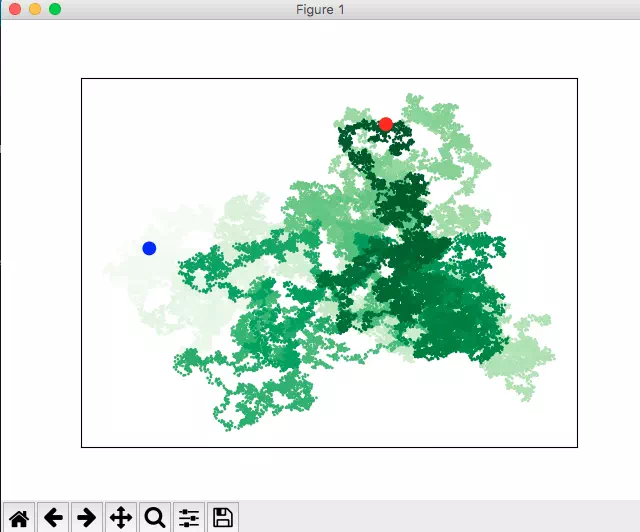# Python Matplotlib Random Walk Example

Random walk means to walk follow a path in a random direction with a random distance from the start point. It is a mathematics concept. Use the python matplotlib module, you can implement random walks easily. This article will tell you how to implement a random walk graph in python.

### 1. Python Matplotlib Random Walk Example

1. Below is this random walk example-generated picture. The blue point is the start point, the red point is the endpoint.2. Below is the example source code, save the source code in a python file then execute it.
```'''
@author: zhaosong
'''

import matplotlib.pyplot
import random

# Generate two random point steps based on the random direction and distance.
def generate_steps(distance_truple=[1,2,3,4,5,6,7,8,9,10]):

# Get random direction.
direction = random.choice([-1,1])

# Get random distance.
distance = random.choice(distance_truple)

step = direction*distance
return step

# Generate all random point x, y value numbers.
def generate_random_walk_list(max_point_numbers):

# Must give a initialize value 0, otherwise the code will throw index out of range error in the while loop.
x_numbers = 
y_numbers = 

# get x value list size.
x_numbers_size = len(x_numbers)

# Loop to create max_point_numbers point coordinate.
while(x_numbers_size < max_point_numbers):
x_step = generate_steps()
y_step = generate_steps()

# If the new point location is same with original, then calculate next point location.
if(x_step==0 and y_step==0):
continue

# Calculate the next x and y by adding new step
next_x = x_numbers[-1] + x_step
next_y = y_numbers[-1] + y_step

# Append new x, y value at the list end.
x_numbers.append(next_x)
y_numbers.append(next_y)

# Recalculate the number list size.
x_numbers_size = len(x_numbers)

# Save the generated x, y value number list in a dictionary to return.
ret = {}
ret['x_numbers'] = x_numbers
ret['y_numbers'] = y_numbers

return ret

# Draw a random walk graph. Each point represent a random position that the walk pass.
def draw_randmon_walk(max_point_numbers):

# Get all random walk points with x, y axis values list.
random_walk_dict = generate_random_walk_list(max_point_numbers)
x_numbers = random_walk_dict['x_numbers']
y_numbers = random_walk_dict['y_numbers']

# Get point color list.
point_color = list(range(max_point_numbers))

# Plot all the point with x, y value based on green colormap.
matplotlib.pyplot.scatter(x_numbers, y_numbers, s=1, c=point_color, cmap=matplotlib.pyplot.cm.Greens)

# Plot the start point as blue.
matplotlib.pyplot.scatter(0, 0, c='blue', edgecolors='none', s=100)

# Plot the end point as red.
matplotlib.pyplot.scatter(x_numbers[-1], y_numbers[-1], c='red', edgecolors='none', s=100)

# Remove all x, y axes.
matplotlib.pyplot.axes().get_xaxis().set_visible(False)
matplotlib.pyplot.axes().get_yaxis().set_visible(False)

# Display the graph.
matplotlib.pyplot.show()

if __name__ == '__main__':
# Plot 50000 random points graph.
draw_randmon_walk(50000)```

Reference

This site uses Akismet to reduce spam. Learn how your comment data is processed.